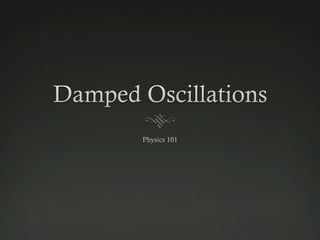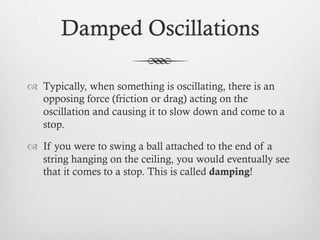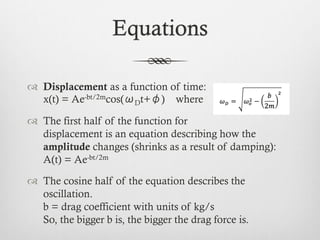Se está descargando tu SlideShare. ×

# Damped Oscillations

Anuncio
Anuncio
Anuncio
Anuncio
Anuncio
Anuncio
Anuncio
Anuncio
Anuncio
Anuncio
AnuncioCargando en…3
×

1 de 9 Anuncio

# Damped Oscillations

A powerpoint for explaining damped oscillations and the equations associated with it. Includes a sample question in the powerpoint. For physics 101 learning object 2.

A powerpoint for explaining damped oscillations and the equations associated with it. Includes a sample question in the powerpoint. For physics 101 learning object 2.

Anuncio
Anuncio

### Damped Oscillations

1. 1. Damped Oscillations   Typically, when something is oscillating, there is an opposing force (friction or drag) acting on the oscillation and causing it to slow down and come to a stop.   If you were to swing a ball attached to the end of a string hanging on the ceiling, you would eventually see that it comes to a stop. This is called damping!
2. 2. Equations   Displacement as a function of time: x(t) = Ae-bt/2mcos(ωDt+φ) where   The first half of the function for displacement is an equation describing how the amplitude changes (shrinks as a result of damping): A(t) = Ae-bt/2m   The cosine half of the equation describes the oscillation. b = drag coefficient with units of kg/s So, the bigger b is, the bigger the drag force is.
3. 3. Frequency   Mass on a spring:   Simple pendulum:   Drag affects frequency, so it’s different in damped oscillations: Natural Frequencies
4. 4. Frequency of Damped Oscillations   This is the equation for frequency in damped oscillations:   Where ωo changes depending on whether you’re working with a simple pendulum or a mass on a spring. You use one of those natural frequencies.
5. 5. Question   Given a system where a mass of 120 g is oscillating on a spring, the spring constant k 97 N/m and the drag coefficient b is 0.18 kg/s, how long will it take for the amplitude to decrease to 25% of its original amplitude? 120 g b=0.18 kg/s k=97 N/m
6. 6. Solution   This is the equation for the displacement of a damped oscillator: x(t) = Ae-bt/2mcos(ωDt+φ)   But since the question is only asking about the amplitude, we only need to use this part of it: A(t) = Ae-bt/2m
7. 7. Solution (cont’d)   The question asks when the amplitude will be 25% of the original, so we go from this: A(t) = Ae-bt/2m to 0.25Ao= Aoe-bt/2m   Here you can see that you don’t actually need to know the initial amplitude because there’s a term on both sides, so they cancel out and you get this: 0.25=e-bt/2m   Since we’re solving for time, we need to bring the power down. We do so by logging both sides.
8. 8. Solution (cont’d)   Logging both sides gives you this: ln0.25 = (-bt/2m)(lne)   The natural log of e is just 1 so you get: ln0.25 = -bt/2m   Now you can isolate t and solve for time:   ln0.25 gives you a negative number, which cancels with the negative in front of the negative 2 to give you a positive number overall. Kg cancel out to give you time in seconds. Notice that k wasn’t used at all. Don’t forget to change from g to kg!